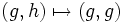# Retraction-invariant subgroup

BEWARE! This term is nonstandard and is being used locally within the wiki. [SHOW MORE]
This article defines a subgroup property: a property that can be evaluated to true/false given a group and a subgroup thereof, invariant under subgroup equivalence. View a complete list of subgroup properties[SHOW MORE]

## Definition

### Symbol-free definition

A subgroup of a group is termed retraction-invariant if any retraction (viz, idempotent endomorphism) of the whole group takes the subgroup to within itself.

### Definition with symbols

A subgroup$H$ of a group$G$ is termed retraction-invariant if, given any retraction (viz, idempotent endomorphism)$\sigma$ of$G$,$\sigma(H) \le H$.

A retraction is an idempotent endomorphism, viz$\sigma$ is a retraction if and only if$\sigma(\sigma(g)) = \sigma(g)$ for all$g$ in$G$.

## Formalisms

BEWARE! This section of the article uses terminology local to the wiki, possibly without giving a full explanation of the terminology used (though efforts have been made to clarify terminology as much as possible within the particular context)

This subgroup property is a monadic second-order subgroup property, viz., it has a monadic second-order description in the theory of groups
View other monadic second-order subgroup properties

### Function restriction expression

This subgroup property is a function restriction-expressible subgroup property: it can be expressed by means of the function restriction formalism, viz there is a function restriction expression for it.
Find other function restriction-expressible subgroup properties | View the function restriction formalism chart for a graphic placement of this property
Function restriction expression$H$ is a retraction-invariant subgroup of$G$ if ... This means that retraction-invariance is ... Additional comments
retraction$\to$ function every retraction of$G$ sends every element of$H$ to within$H$ the invariance property for retractions
retraction$\to$ endomorphism every retraction of$G$ restricts to an endomorphism of$H$ the endo-invariance property for retractions; i.e., it is the invariance property for retraction, which is a property stronger than the property of being an endomorphism
retraction$\to$ retraction every retraction of$G$ restricts to a retraction of$H$ the balanced subgroup property for retractions Hence, it is a t.i. subgroup property, both transitive and identity-true

## Relation with other properties

### Weaker properties

• Direct projection-invariant subgroup
• Retract-transfering subgroup: In other words, if$H$ is a retraction-invariant subgroup of$G$ and$K$ is a retract of$G$, then$H \cap K$ is a retract of$H$.

### Incomparable with normality

Note that there are retraction-invariant subgroups which are not normal. In fact, in a simple group, every subgroup is retraction-invariant, although none except the trivial subgroup and the whole group are normal.

Further, we have examples of normal subgroups that are not retraction-invariant. For instance, the copy of$G$ in$G \times G$ is not invariant under the retraction$(g,h) \mapsto (g,g)$.

## Metaproperties

### Transitivity

This subgroup property is transitive: a subgroup with this property in a subgroup with this property, also has this property in the whole group.
ABOUT THIS PROPERTY: View variations of this property that are transitive | View variations of this property that are not transitive
ABOUT TRANSITIVITY: View a complete list of transitive subgroup properties|View a complete list of facts related to transitivity of subgroup properties |Read a survey article on proving transitivity

Any retraction-invariant subgroup of a retraction-invariant subgroup is retraction-invariant. This easily follows on account of retraction-invariance being a balanced subgroup property, that is, from the fact that its restriction formal expression has the same thing on the left side and on the right side.

### Trimness

This subgroup property is trim -- it is both trivially true (true for the trivial subgroup) and identity-true (true for a group as a subgroup of itself).
View other trim subgroup properties | View other trivially true subgroup properties | View other identity-true subgroup properties

The whole group and the trivial subgroup are clearly retraction-invariant.

### Intersection-closedness

YES: This subgroup property is intersection-closed: an arbitrary (nonempty) intersection of subgroups with this property, also has this property.
ABOUT THIS PROPERTY: View variations of this property that are intersection-closed | View variations of this property that are not intersection-closed
ABOUT INTERSECTION-CLOSEDNESS: View all intersection-closed subgroup properties (or, strongly intersection-closed properties) | View all subgroup properties that are not intersection-closed | Read a survey article on proving intersection-closedness | Read a survey article on disproving intersection-closedness

An arbitrary intersection of retraction-invariant subgroups is retraction-invariant. This follows from its being an invariance property. For full proof, refer: Invariance implies intersection-closed

### Join-closedness

YES: This subgroup property is join-closed: an arbitrary (nonempty) join of subgroups with this property, also has this property.
ABOUT THIS PROPERTY: View variations of this property that are join-closed | View variations of this property that are not join-closed
ABOUT JOIN-CLOSEDNESS: View all join-closed subgroup properties (or, strongly join-closed properties) | View all subgroup properties that are not join-closed | Read a survey article on proving join-closedness | Read a survey article on disproving join-closedness

An arbitrary join of retraction-invariant subgroups is retraction-invariant. This follows from its being an endo-invariance property. For full proof, refer: Endo-invariance implies join-closed